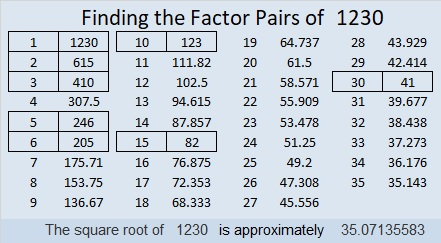# 1230 Mystery

Is this mystery level puzzle easy or difficult? The only way to know for sure is to start filling in the factors. Don’t guess and check. Use logic to find its unique solution!Print the puzzles or type the solution in this excel file: 10-factors-1221-1231

Here is some information about the number 1230:

1230 ends with a zero so it is divisible by 2 and 5.
It is a number formed by three consecutive numbers and a zero so it is divisible by 3.

• 1230 is a composite number.
• Prime factorization: 1230 = 2 × 3 × 5 × 41
• The exponents in the prime factorization are 1, 1, 1, and 1. Adding one to each and multiplying we get (1 + 1)(1 + 1)(1 + 1)(1 + 1) = 2 × 2 × 2 × 2 = 16. Therefore 1230 has exactly 16 factors.
• Factors of 1230: 1, 2, 3, 5, 6, 10, 15, 30, 41, 82, 123, 205, 246, 410, 615, 1230
• Factor pairs: 1230 = 1 × 1230, 2 × 615, 3 × 410, 5 × 246, 6 × 205, 10 × 123, 15 × 82, or 30 × 41
• 1230 has no square factors that allow its square root to be simplified. √1230 ≈ 35.071361230 is the hypotenuse of four Pythagorean triples:
270-1200-1230 which is 30 times (9-40-41)
504-1122-1230 which is 6 times (84-187-205)
738-984-1230 which is (3-4-5) times 246
798-936-1230 which is 6 times (133-156-205)

This site uses Akismet to reduce spam. Learn how your comment data is processed.